Courses

# NCERT Exemplar: Quadratic Equations Notes | EduRev

## Class 10 : NCERT Exemplar: Quadratic Equations Notes | EduRev

The document NCERT Exemplar: Quadratic Equations Notes | EduRev is a part of the Class 10 Course Mathematics (Maths) Class 10.
All you need of Class 10 at this link: Class 10

Exercise 4.1

Choose the correct answer from the given four options in the following questions:
1. Which of the following is a quadratic equation?
(a) x2 + 2x + 1 = (4 – x)2 + 3
(b) –2x2 = (5 – x)(2x-(2/5))
(c) (k + 1) x2 + (3/2) x = 7, where k = –1
(d) x3 – x2 = (x – 1)3
Ans: (d)
Explanation:
The standard form of a quadratic equation is given by,
ax2 + bx + c = 0, a ≠ 0
(A) Given, x2 + 2x + 1 = (4 – x)2 + 3
x2 + 2x + 1 = 16 – 8x + x2 + 3
10x – 18 = 0
which is not a quadratic equation.
(B) Given, -2x2 = (5 – x) (2x – 2/5)
-2x2 = 10x – 2x2 – 2 +2/5x
52x – 10 = 0
which is not a quadratic equation.
(C) Given, (k + 1) x2 + 3/2 x  = 7, where k = -1
(-1 + 1) x2 + 3/2 x = 7
3x – 14 = 0
which is not a quadratic equation.
(D) Given, x3 – x2 = (x – 1)3
x3 – x2 = x3 – 3x2 + 3x – 1
2x2 – 3x + 1 = 0
which represents a quadratic equation.

2. Which of the following is not a quadratic equation?
(a) 2(x – 1)2 = 4x2 – 2x + 1
(b) 2x – x2 = x2 + 5
(c) (2x + 3)2 + x2 = 3x2 − 5x
(d) (x2 + 2x)2 = x4 + 3 + 4x3

Ans: (d)
Explanation:
A quadratic equation is represented by the form,
ax2 + bx + c = 0, a ≠ 0
(A) Given, 2(x – 1)2 = 4x2 – 2x + 1
2(x2 – 2x + 1) = 4x2 – 2x + 1
2x2 + 2x – 1 = 0
which is a quadratic equation.
(B) Given, 2x – x2 = x2 + 5
2x2 – 2x + 5 = 0
which is a quadratic equation.
(C) Given, (√2x + √3)2  = 3x2 – 5x
2x2 + 2√6x + 3  = 3x2 – 5x
x2 – (5 + 2√6)x – 3 = 0
which is a quadratic equation.
(D) Given, (x2 + 2x)2 = x4 + 3 + 4x2
x4 + 4x3 + 4x2 = x4 + 3 + 4x2
4x3 – 3 = 0
which is a cubic equation and not a quadratic equation.

3. Which of the following equations has 2 as a root?
(a) x2 – 4x + 5 = 0
(b) x2 + 3x – 12 = 0
(c) 2x2 – 7x + 6 = 0
(d) 3x2 – 6x – 2 = 0

Ans: (c)
Explanation:
If 2 is a root then substituting the value 2 in place of x should satisfy the equation.
(A) Given,
x2 – 4x + 5 = 0
(2)2 – 4(2) + 5 = 1 ≠ 0
So, x = 2 is not a root of x2 – 4x + 5 = 0
(B) Given, x2 + 3x – 12 = 0
(2)2 + 3(2) – 12 = -2 ≠ 0
So, x = 2 is not a root of x2 + 3x – 12 = 0
(C) Given, 2x2 – 7x + 6 = 0
2(2)2 – 7(2) + 6 = 0
Here, x = 2 is a root of 2x2 – 7x + 6 = 0
(D) Given, 3x2 – 6x – 2 = 0
3(2)2 – 6(2) – 2 = -2 ≠ 0
So, x = 2 is not a root of 3x2 – 6x – 2 = 0

4. If ½ is a root of the equation x2 + kx – 5/4 = 0, then the value of k is
(A) 2
(B) – 2
(C) ¼
(D) ½
Ans: (a)
Explanation:
If ½ is a root of the equation
x2 + kx – 5/4 = 0 then, substituting the value of ½ in place of x should give us the value of k.
Given, x2 + kx – 5/4 = 0 where, x = ½
(½)2 + k (½) – (5/4) = 0
(k/2) = (5/4) – ¼
k = 2

5. Which of the following equations has the sum of its roots as 3?
(a) 2x2 – 3x + 6 = 0
(b) –x2 + 3x – 3 = 0

(c) √2x– 3/√2x + 1 = 0
(d) 3x2 – 3x + 3 = 0
Ans: (b)
Explanation:

The sum of the roots of a quadratic equation ax2 + bx + c = 0, a ≠ 0 is given by,
Coefficient of x / coefficient of x2 = – (b/a)
(A) Given, 2x2 – 3x + 6 = 0
Sum of the roots = – b/a = -(-3/2) = 3/2
(B) Given, -x2 + 3x – 3 = 0
Sum of the roots = – b/a = -(3/-1) = 3
(C) Given, √2x– 3/√2x+1=0
2x2 – 3x + √2 = 0
Sum of the roots = – b/a = -(-3/2) = 3/2
(D) Given, 3x2 – 3x + 3 = 0
Sum of the roots = – b/a = -(-3/3) = 1

Exercise 4.2

1. State whether the following quadratic equations have two distinct real roots. Justify your answer.

(i) x2 – 3x + 4 = 0

(ii) 2x2 + x – 1 = 0

(iii) 2x2 – 6x + 9/2 = 0

(iv) 3x2 – 4x + 1 = 0

(v) (x + 4)2 – 8x = 0

(vi) (x – 2)2 – 2(x + 1) = 0

(vii) 2 x2 –(3/√2)x + 1/√2 = 0

(viii) x (1 – x) – 2 = 0

(ix) (x – 1) (x + 2) + 2 = 0

(x) (x + 1) (x – 2) + x = 0
Solution:
(i)
The equation x2 – 3x + 4 = 0 has no real roots.
D = b2 – 4ac
= (-3)2 – 4(1)(4)
= 9 – 16 < 0
Hence, the roots are imaginary.
(ii)
The equation 2x2 + x – 1 = 0 has two real and distinct roots.
D = b2 – 4ac
= 12 – 4(2) (-1)
= 1 + 8 > 0
Hence, the roots are real and distinct.
(iii)
The equation 2x2 – 6x + (9/2) = 0 has real and equal roots.
D = b2 – 4ac
= (-6)2 – 4(2) (9/2)
= 36 – 36 = 0
Hence, the roots are real and equal.
(iv)
The equation 3x2 – 4x + 1 = 0 has two real and distinct roots.
D = b2 – 4ac
= (-4)2 – 4(3)(1)
= 16 – 12 > 0
Hence, the roots are real and distinct.
(v)
The equation (x + 4)2 – 8x = 0 has no real roots.
Simplifying the above equation,
x2 + 8x + 16 – 8x = 0
x2 + 16 = 0
D = b2 – 4ac
= (0) – 4(1) (16) < 0
Hence, the roots are imaginary.
(vi)
The equation (x – √2)– √2(x+1)=0 has two distinct and real roots.
Simplifying the above equation,
x2 – 2√2x + 2 – √2x – √2 = 0
x2 – √2(2+1)x + (2 – √2) = 0
x2 – 3√2x + (2 – √2) = 0
D = b2 – 4ac
= (– 3√2)2 – 4(1)(2 – √2)
= 18 – 8 + 4√2 > 0
Hence, the roots are real and distinct.
(vii)
The equation √2x2 – 3x/√2 + ½ = 0 has two real and distinct roots.
D = b2 – 4ac
= (- 3/√2)2 – 4(√2) (½)
= (9/2) – 2√2 > 0
Hence, the roots are real and distinct.
(viii)
The equation x (1 – x) – 2 = 0 has no real roots.
Simplifying the above equation,
x2 – x + 2 = 0
D = b2 – 4ac
= (-1)2 – 4(1)(2)
= 1 – 8 < 0
Hence, the roots are imaginary.
(ix)
The equation (x – 1) (x + 2) + 2 = 0 has two real and distinct roots.
Simplifying the above equation,
x2 – x + 2x – 2 + 2 = 0
x2 + x = 0
D = b2 – 4ac
= 12 – 4(1)(0)
= 1 – 0 > 0
Hence, the roots are real and distinct.
(x)
The equation (x + 1) (x – 2) + x = 0 has two real and distinct roots.
Simplifying the above equation,
x2 + x – 2x – 2 + x = 0
x2 – 2 = 0
D = b2 – 4ac
= (0)2 – 4(1) (-2)
= 0 + 8 > 0
Hence, the roots are real and distinct.

2. Write whether the following statements are true or false. Justify your answers.

(i) Every quadratic equation has exactly one root.

(ii) Every quadratic equation has at least one real root.

(iii) Every quadratic equation has at least two roots.

(iv) Every quadratic equations has at most two roots.

(v) If the coefficient of x2 and the constant term of a quadratic equation have opposite signs, then the quadratic equation has real roots.

(vi) If the coefficient of x2 and the constant term have the same sign and if the coefficient of x term is zero, then the quadratic equation has no real roots.
Solution:
(i) False. For example, a quadratic equation x2 – 9 = 0 has two distinct roots – 3 and 3.
(ii) False. For example, equation x2 + 4 = 0 has no real root.
(iii) False. For example, a quadratic equation x2 – 4+ 4 = 0 has only one root which is 2.
(iv) True, because every quadratic polynomial has almost two roots.
(v) True, because in this case discriminant is always positive.
For example, in ax2+ bx + c = 0, as a and c have opposite sign, ac < 0
⟹ Discriminant = b2 – 4ac > 0.
(vi) True, because in this case discriminant is always negative.
For example, in ax2+ bx + c = 0, as b = 0, and a and c have same sign then ac > 0
⟹ Discriminant = b2 – 4ac = – 4 a c < 0

3. A quadratic equation with integral coefficient has integral roots. Justify your answer.
Solution:

No, a quadratic equation with integral coefficients may or may not have integral roots.
Justification
Consider the following equation,
8x2 – 2x – 1 = 0
The roots of the given equation are ½ and – ¼ which are not integers.
Hence, a quadratic equation with integral coefficient might or might not have integral roots.

Exercise 4.3

1. Find the roots of the quadratic equations by using the quadratic formula in each of the following:

(i) 2 x2 – 3x – 5 = 0

(ii) 5x2 + 13x + 8 = 0

(iii) –3x2 + 5x + 12 = 0

(iv) –x2 + 7x – 10 = 0

(v) x2 + 22x – 6 = 0

(vi) x2 – 35x + 10 = 0

(vii) (½)x2– √11x + 1 = 0
Solution:
The quadratic formula for finding the roots of quadratic equation
ax2 + bx + c = 0, a ≠ 0 is given by,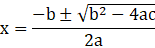(i) 2 x2 – 3x – 5 = 0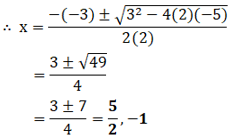(ii) 5x2 + 13x + 8 = 0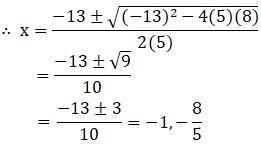(iv) –x2 + 7x – 10 = 0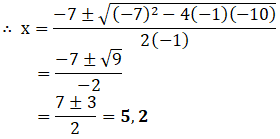(v) x2 + 2 √2x – 6 = 0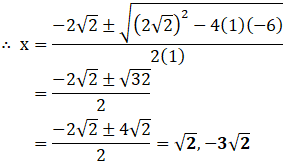(vi) x2 – 3 √5x + 10 = 0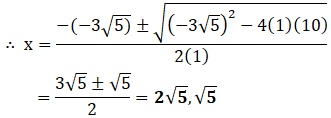(vii) (½)x2– √11x + 1 = 0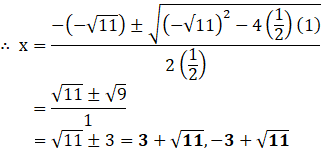Exercise 4.4

1. Find a natural number whose square diminished by 84 is equal to thrice of 8 more than the given number.
Solution:
Let the natural number = ‘x’.
According to the question,
We get the equation,
x² – 84 = 3(x + 8)
x² – 84 = 3x + 24
x² – 3x – 84 – 24 = 0
x² – 3x – 108 = 0
x² – 12x + 9x – 108 = 0
x(x – 12) + 9(x – 12) = 0
(x + 9) (x – 12)
⇒ x = -9 and x = 12
Since, natural numbers cannot be negative.
The number is 12.

2. A natural number, when increased by 12, equals 160 times its reciprocal. Find the number.
Solution:
Let the natural number = x
When the number increased by 12 = x + 12
Reciprocal of the number = 1/x
According to the question, we have,
x + 12 = 160 times of reciprocal of x
x + 12 = 160/ x
x( x + 12 ) = 160
x2 + 12x – 160 = 0
x2 + 20x – 8x – 160 = 0
x( x + 20) – 8( x + 20)=  0
(x + 20) (x – 8) = 0
x + 20 = 0 or x – 8 = 0
x = – 20 or x = 8
Since, natural numbers cannot be negative.
The required number = x = 8

3. A train, travelling at a uniform speed for 360 km, would have taken 48 minutes less to travel the same distance if its speed were 5 km/h more. Find the original speed of the train.
Solution:
Let original speed of train = x km/h
We know,
Time = distance/speed
According to the question, we have,
Time taken by train = 360/x hour
And, Time taken by train its speed increase 5 km/h = 360/( x + 5)
It is given that,
Time taken by train in first – time taken by train in 2nd case = 48 min = 48/60 hour
360/x – 360/(x +5) = 48/60 = 4/5
360(1/x – 1/(x +5)) = 4/5
360 ×5/4 (5/(x² +5x)) =1
450 × 5 = x² + 5x
x² +5x -2250 = 0
x = (-5± √ (25+9000))/2
= (-5 ±√ (9025) )/2
= (-5 ± 95)/2
= -50, 45
But x ≠ -50 because speed cannot be negative
So, x = 45 km/h
Hence, original speed of train = 45 km/h

4. If Zeba were younger by 5 years than what she really is, then the square of her age (in years) would have been 11 more than five times her actual age. What is her age now?
Solution:
Let Zeba’s age = x
According to the question,
(x-5)²=11 + 5x
x² + 25 - 10x = 11 + 5x
x² - 15x + 14 = 0
x² - 14x - x + 14 = 0
x(x -14) -1(x - 14) = 0
x = 1 or x = 14
We have to neglect 1 as 5 years younger than 1 cannot happen.
Therefore, Zeba’s present age = 14 years.

Offer running on EduRev: Apply code STAYHOME200 to get INR 200 off on our premium plan EduRev Infinity!

## Mathematics (Maths) Class 10

51 videos|346 docs|103 tests

,

,

,

,

,

,

,

,

,

,

,

,

,

,

,

,

,

,

,

,

,

;